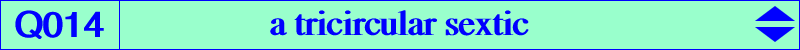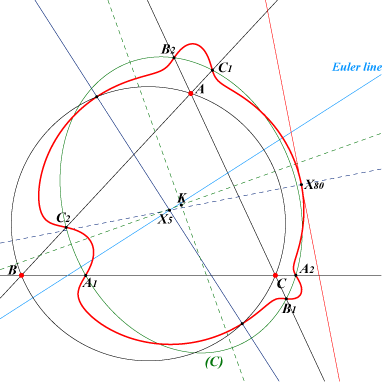too complicated to be written here. Click on the link to download a text file.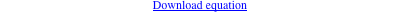X(80) and its extraversions other points belowQ014 is the locus of singularities of cK60+ cubics (see Special Isocubics §8.6). See for example K230. Q014 is a tricircular sextic with A, B, C double points. It meets the sidelines of ABC again at six points A1, A2, B1, B2, C1, C2 lying on a conic (C) with center K = X(6), with axes parallel to the asymptotes of the Jerabek hyperbola. This conic is an ellipse when ABC is acutangle, a hyperbola when ABC is obtusangle. The tangents at A1, A2 pass through A, the other tangents similarly. Notice that the midpoint of A1A2 is the foot of the altitude AH. Q014 contains X(80) with a tangent perpendicular to the line X(5)X(80) and the two common points of the perpendicular at X(5) to the Euler line and the circumcircle. Locus property Let P be a point and La, Lb, Lc the reflections of the sidelines of ABC in AP, BP, CP respectively. La, Lb, Lc concur if and only if P lies on Q014 (Angel Montesdeoca, 2019-12-05).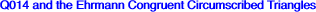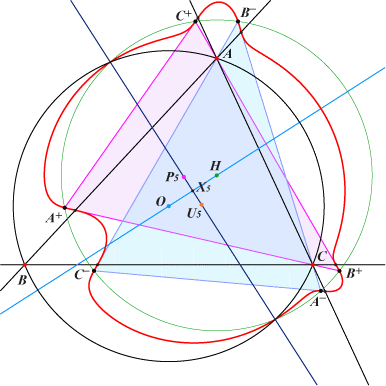(contributed by Richard Hilton on 2011-06-23) Jean-Pierre Ehrmann showed that for any triangle ABC there are precisely two triangles that are congruent to ABC and circumscribe it. These are formed by rotations of ABC by ±120° about the bicentric pair of Ehrmann pivots listed by Kimberling as P(5) and U(5) respectively. These are the points denoted by A±, B±, C± on the figure. The sextic Q014 passes through the vertices of these triangles, as well as (tangentially) through the intersections of their sides with the corresponding sides of ABC. These are the points A1, A2, B1, B2, C1, C2 above. The barycentric coordinates of the vertex A+ are : (SB – K)(SC + K) : (SC + K)(SA + K) : (SA – K)(SB – K), using Conway’s notation and where K = 2Δ / √3 and Δ is the area of ABC. For the other vertex A– the coordinates are : (SB + K)(SC – K) : (SC – K)(SA – K) : (SA + K)(SB + K).The points of intersection A1, A2 with BC have coordinates 0 : SC + K : SB – K and 0 : SC – K : SB + K. Remarks : the line through A+ and A– meets BC on the line through X(5) X(523) P(5) U(5), the lines BC, B+C– and B–C+ are parallel, the barycentric product of A+ and A–, B+ and B–, C+ and C– is X(1989) the six points A±, B±, C± lie on the circle C(H, R) and on a cubic nK with pole X(1989), root X(2).